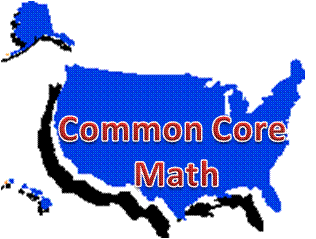# Common Core High School Statistics & Probability Teaching and Learning Resources

## Domain: IC: Making Inferences and Justifying Conclusions• IC-A: Understand and evaluate random processes underlying statistical experiments

• IC-B: Make inferences and justify conclusions from sample surveys, experiments, and observational studies### IC-A: Understand and evaluate random processes underlying statistical experiments

Standards:

• IC-A.1. Understand statistics as a process for making inferences about population parameters based on a random sample from that population.
• IC-A.2. Decide if a specified model is consistent with results from a given data-generating process, e.g., using simulation. For example, a model says a spinning coin falls heads up with probability 0.5. Would a result of 5 tails in a row cause you to question the model?

Technology-enhanced investigations:

Stattrek.com Statistics Dictionary contains the following key vocabulary for this domain.  Select the term from the Stattrek pull-down menu.  Use with IC-A, IC-B:

 experiment observational study randomization significance level margin of error parameter random sample statistics mean population sample survey treatment

LearnZillion:

MIT BLOSSOMS: Video lessons with additional teacher and learner resources.  Descriptions are from the video summaries.

• Flu Math Games: "This video lesson shows students that math can play a role in understanding how an infectious disease spreads and how it can be controlled."  Additional simulations are included.  Aligns with Algebra standards SSE-B.3.c and REI-A.1; Function standards IF-C.8.b, BF-B.4.a, and LE-A-1.(a, c); and Statistics and Probability standards ID-B.6.a, IC-A.1, IC-B.4, CP-A.2, and MD-A.1.
• Averages: Still Flawed: "This learning video continues the theme of an early BLOSSOMS lesson, Flaws of Averages, using new examples—including how all the children from Lake Wobegon can be above average, as well as the Friendship Paradox. As mentioned in the original module, averages are often worthwhile representations of a set of data by a single descriptive number. The objective of this module, once again, is to simply point out a few pitfalls that could arise if one is not attentive to details when calculating and interpreting averages."  Aligns with IC-A.2.

PhET Interactive Simulations: Plinko Probability. Per its description: "Drop balls through a triangular grid of pegs and see them accumulate in containers. Switch to a histogram view and compare the distribution of balls to an ideal binomial distribution. Adjust the binary probability and develop your knowledge of statistics!"  Aligns with ID-A.1, ID-A.2, and IC-A.2.

Stat Trek: Tutorial: Simulation of Random Events

Wolfram Demonstrations Project: Download the free Wolfram CDF player to interact with the following manipulatives.  Note: Within the Wolfram Demonstration Project are 7 manipulatives addressing IC-A.1 and 2 manipulatives addressing IC-A.2:  Among those are:

Multiple Choice:

Khan Academy: Significance Tests (Hypothesis testing) unit. Practice questions with videos.

Constructed-response:

Illustrative Mathematics: Statistics and Probability:

Mathematics Vision Project, Secondary 3 Student Edition:

NCTM's Reasoning and Sense Making Task Library: Eruptions: Old Faithful includes the task overview, teacher notes for its use in the classroom, and student activity sheet.  Aligns with IC-A.1, ID-A.1, and mathematical practice standards 1, 3, and 5.

Statistics in Schools from the U.S. Census Bureau: Activities: Math: 9-12:

• Educational Attainment and Marriage: Testing a Correlation Coefficient's Significance.  Students "develop, justify, and evaluate conjectures about the relationship between two quantitative variables over time in the United States: the median age (in years) when women first marry and the percentage of women aged 25–34 with a bachelor’s degree or higher. Students will write a regression equation for the data, interpret in context the linear model’s slope and y-intercept, and find the correlation coefficient (r), assessing the strength of the linear relationship and whether a significant relationship exists between the variables. Students will then summarize their conclusions and consider whether correlation implies causation."  Aligns with standards ID-C.8, ID-C.9, and IC-A.1.

### IC-B: Make inferences and justify conclusions from sample surveys, experiments, and observational studies

Standards:

• IC-B.3. Recognize the purposes of and differences among sample surveys, experiments, and observational studies; explain how randomization relates to each.
• IC-B.4. Use data from a sample survey to estimate a population mean or proportion; develop a margin of error through the use of simulation models for random sampling.
• IC-B.5. Use data from a randomized experiment to compare two treatments; use simulations to decide if differences between parameters are significant.
• IC-B.6. Evaluate reports based on data.

Technology-enhanced investigations:

Khan Academy: Videos: Bernoulli distributions and margins of error.  Use with IC-B.4.

LearnZillion:

MIT BLOSSOMS: Video lesson with additional teacher and learner resources.  Description is from the video summary.  Flu Math Games: "This video lesson shows students that math can play a role in understanding how an infectious disease spreads and how it can be controlled."  Additional simulations are included.  Aligns with Algebra standards SSE-B.3.c and REI-A.1; Function standards IF-C.8.b, BF-B.4.a, and LE-A-1.(a, c); and Statistics nd Probability standards ID-B.6.a, IC-A.1, IC-B.4, CP-A.2, and MD-A.1.

Shodor Interactivate: Statistics and Probability Making Inferences and Justifying Conclusions: Understand and evaluate random processes underlying statistical experiments.  This includes 10 lessons and 22 activities with virtual manipulatives involving simulations and experiments.

Statistics How To: Misleading Graphs: Real Life Examples

Stat Trek: Tutorials on Surveys and Experiments: Surveys: Data Collection Methods: This tutorial presents four main methods of data collection: census, sample surveys, experiments, and observational studies; What is an experiment?; and Experimental Design in Statistics

Wolfram Demonstrations Project: Download the free Wolfram CDF player to interact with the following manipulatives.  Note: Within the Wolfram Demonstration Project are 1 manipulative addressing IC-B.3, 3 manipulatives addressing IC-B.4, and 2 manipulatives addressing IC-B.5. Among those are:

Multiple Choice:

Khan Academy: Practice questions with videos.

Constructed-response:

Illustrative Mathematics: Statistics and Probability:

Mathematics Assessment Project: Standards: High School: Statistics & Probability: Task 217: Interpreting Statistics: A Case of Muddying the Waters

Mathematics Vision Project, Secondary 3 Student Edition: